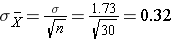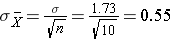# Central Limit Theorem with a Skewed Distribution

## Central Limit Theorem with a Skewed Distribution

The Poisson distribution is another probability model that is useful for modeling discrete variables such as the number of events occurring during a given time interval. For example, suppose you typically receive about 4 spam emails per day, but the number varies from day to day. Today you happened to receive 5 spam emails. What is the probability of that happening, given that the typical rate is 4 per day? The Poisson probability is: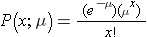Mean = μ

Standard deviation =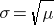The mean for the distribution is μ (the average or typical rate), “X” is the actual number of events that occur (“successes”), and “e” is the constant approximately equal to 2.71828. So, in the example above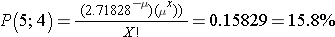Now let’s consider another Poisson distribution. with μ=3 and σ=1.73. The distribution is shown in the figure below.

This population is not normally distributed, but the Central Limit Theorem will apply if n > 30. In fact, if we take samples of size n=30, we obtain samples distributed as shown in the first graph below with a mean of 3 and standard deviation = 0.32. In contrast, with small samples of n=10, we obtain samples distributed as shown in the lower graph. Note that n=10 does not meet the criterion for the Central Limit Theorem, and the small samples on the right give a distribution that is not quite normal. Also note that the sample standard deviation (also called the “standard error”) is larger with smaller samples, because it is obtained by dividing the population standard deviation by the square root of the sample size. Another way of thinking about this is that extreme values will have less impact on the sample mean when the sample size is large.